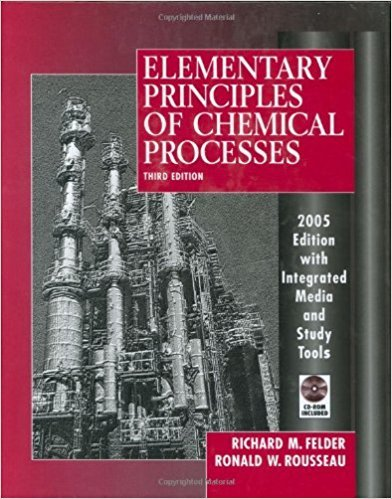×
Get Full Access to Elementary Principles Of Chemical Processes - 3 Edition - Chapter 7 - Problem 7.49
Get Full Access to Elementary Principles Of Chemical Processes - 3 Edition - Chapter 7 - Problem 7.49

×

# A perfectly insulated cylinder fitted with a leakproofISBN: 9780471687573 143

## Solution for problem 7.49 Chapter 7

Elementary Principles of Chemical Processes | 3rd Edition

• Textbook Solutions
• 2901 Step-by-step solutions solved by professors and subject experts
• Get 24/7 help from StudySoup virtual teaching assistantsElementary Principles of Chemical Processes | 3rd Edition

4 5 1 292 Reviews
17
2
Problem 7.49

A perfectly insulated cylinder fitted with a leakproof frictionless piston with a mass of 30.0 kg and a face area of 400.0 cm2 contains 7.0 kg of liquid water and a 3.0-kg bar of aluminum. The aluminum bar has an electrical coil imbedded in it, so that known amounts of heat can be transferred to it. Aluminum has a specific gravity of 2.70 and a specific internal energy given by the formula O(kJ/kg) = 0.94TCc). The internal energy of liquid water at any temperature may be taken to be that of the saturated liquid at that temperature. Negligible heat is transferred to the cylinder wall. Atmospheric pressure is 1.00 atm. The cylinder and its contents are initially at 20C. m = 30 kg ~~-'-......,~ .. A = 400 cm2 H20(v) 3 kg AI Q H20(1) Suppose that 3310 kJ is transferred to the bar from the heating coil and the contents of the cylinder are then allowed to equilibrate. (a) Calculate the pressure of the cylinder contents throughout the process. Then determine whether the amount of heat transferred to the system is sufficient to vaporize any of the water. (b) Determine the following quantities: (i) the final system temperature; (ii) the volumes (cm3) of the liquid and vapor phases present at equilibrium; and (iii) the vertical distance traveled by the piston from the beginning to the end of the process. [Suggestion: Write an energy balance on the complete process, taking the cylinder contents to be the system. Note that the system is closed and that work is done by the system when it moves the piston through a vertical displacement. The magnitude of this work is W = P L\V, where P is the constant system pressure and L\V is the change in system volume from the initial to the final state.] (c) Calculate an upper limit on the temperature attainable by the aluminum bar during the process, and state the condition that would have to apply for the bar to come close to this temperature.

Step-by-Step Solution:
Step 1 of 3

Step 2 of 3

Step 3 of 3

##### ISBN: 9780471687573

This full solution covers the following key subjects: system, cylinder, temperature, bar, process. This expansive textbook survival guide covers 13 chapters, and 710 solutions. Since the solution to 7.49 from 7 chapter was answered, more than 566 students have viewed the full step-by-step answer. The answer to “A perfectly insulated cylinder fitted with a leakproof frictionless piston with a mass of 30.0 kg and a face area of 400.0 cm2 contains 7.0 kg of liquid water and a 3.0-kg bar of aluminum. The aluminum bar has an electrical coil imbedded in it, so that known amounts of heat can be transferred to it. Aluminum has a specific gravity of 2.70 and a specific internal energy given by the formula O(kJ/kg) = 0.94TCc). The internal energy of liquid water at any temperature may be taken to be that of the saturated liquid at that temperature. Negligible heat is transferred to the cylinder wall. Atmospheric pressure is 1.00 atm. The cylinder and its contents are initially at 20C. m = 30 kg ~~-'-......,~ .. A = 400 cm2 H20(v) 3 kg AI Q H20(1) Suppose that 3310 kJ is transferred to the bar from the heating coil and the contents of the cylinder are then allowed to equilibrate. (a) Calculate the pressure of the cylinder contents throughout the process. Then determine whether the amount of heat transferred to the system is sufficient to vaporize any of the water. (b) Determine the following quantities: (i) the final system temperature; (ii) the volumes (cm3) of the liquid and vapor phases present at equilibrium; and (iii) the vertical distance traveled by the piston from the beginning to the end of the process. [Suggestion: Write an energy balance on the complete process, taking the cylinder contents to be the system. Note that the system is closed and that work is done by the system when it moves the piston through a vertical displacement. The magnitude of this work is W = P L\V, where P is the constant system pressure and L\V is the change in system volume from the initial to the final state.] (c) Calculate an upper limit on the temperature attainable by the aluminum bar during the process, and state the condition that would have to apply for the bar to come close to this temperature.” is broken down into a number of easy to follow steps, and 336 words. Elementary Principles of Chemical Processes was written by and is associated to the ISBN: 9780471687573. The full step-by-step solution to problem: 7.49 from chapter: 7 was answered by , our top Chemistry solution expert on 11/15/17, 02:42PM. This textbook survival guide was created for the textbook: Elementary Principles of Chemical Processes, edition: 3.

Unlock Textbook Solution